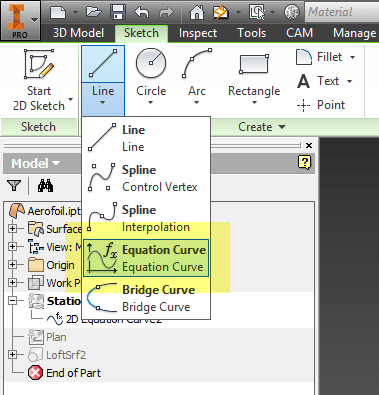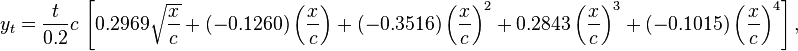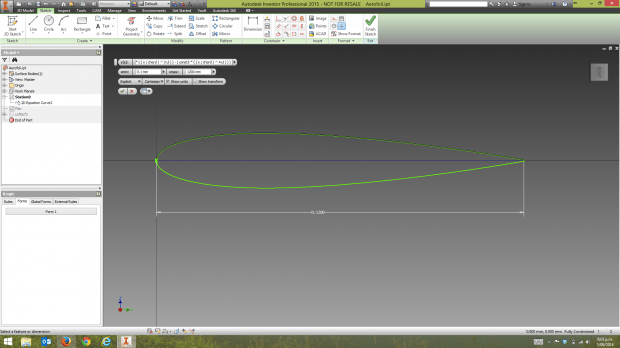Equation Curve Command in Inventor’s Sketch Environment

The 2D equation curve function has been available in the Inventor sketch environment for a few releases now, but I haven’t seen all that much written about it, and don’t know too many people who are using it. For those reasons, I thought I’d do a quick post with an example of it’s use.

## Background

Before it effectively became NASA in 1958, NACA (National Advisory Commitee for Aeonautics) was a U.S. Federal Agency that was set up to undertake, promote and institutionalize aeronautical research. One of the important results of their research was the development of a series of aerofoil profiles, in the 1940s, that are still in use in aircraft and marine design today. A NACA aerofoil is one whose shape is determined by one of a series of mathematical equations. In this example, I used the “4-digit” symmetrical equation to create a non-cambered aerofoil. My next challenge is to create a cambered profile using the relevant equation.

## The Math

The following is an excerpt from this wikipedia entry:

### Equation for a symmetrical 4-digit NACA airfoil

The formula for the shape of a NACA 00xx foil, with “xx” being replaced by the percentage of thickness to chord, is:$y_t = \frac{t}{0.2}c\, \left[ 0.2969 \sqrt{\frac{x}{c}} + (-0.1260) \left(\frac{x}{c}\right) + (-0.3516) \left(\frac{x}{c}\right)^2 + 0.2843 \left(\frac{x}{c}\right)^3 + (-0.1015) \left( \frac{x}{c} \right)^4 \right],$

where:

• c is the chord length,
• x is the position along the chord from 0 to c,
• y is the half thickness at a given value of x (centerline to surface), and
• t is the maximum thickness as a fraction of the chord (so 100 t gives the last two digits in the NACA 4-digit denomination).

## The Model

I created a 2D sketch in Inventor 2015, and used the equation curve tool (hidden in the “Line” dropdown menu) to input the formula above.2D Equation Curve in Inventor Sketch Environment

If you’d like to recreate this, here is the formula in a copy-paste-able format:

( ( t / 0.2 ul ) * chord ) * ( ( const1 * sqrt(x / chord) ) – ( const2 * ( x / chord ) ) – ( const3 * ( ( x / chord ) ^ 2 ul ) ) + ( const4 * ( ( x / chord ) ^ 3 ul ) ) – ( const5 * ( ( x / chord ) ^ 4 ul ) ) )

The equation only creates one half of the curve, so I used the mirror tool with the “Self Symmetric” option ticked to get the leading edge. The trailing edge is a very short vertical line.

## The iLogic (of course there is iLogic, it’s me, iGav)

It’s all well and good to have a static aerofoil section for an example, but for any practical application, you’d want to be able to adjust the shape and parameters to get exactly the shape you require. An iLogic form provides a really easy-to-use interface for adjusting the chord length and thickness of the section.Aerofoil Parameters can be Adjusted with an iLogic Form

While I was there, I thought I’d add a wing plan sketch with some control planes and a series of stations. This turns the aerofoil section into a wing shape and allows me to adjust the leadin and trailing edge taper angles.

If you’re interested, here’s the Inventor 2015 model file: Aerofoil iLogic Model

If any of you have a particular equation for something interesting, that you’d like to see applied to a model, please get in touch.

Feature image credit: simpleinsomnia – “Students study an equation on a chalk board” – Unedited

[subscribe2]